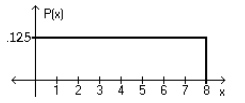Bài sắp đăng
Home | Trắc nghiệm | Kinh tế lượng | 500 câu trắc nghiệm Kinh tế lượng – 5CTổng hợp 500 câu trắc nghiệm + tự luận Kinh tế lượng (Elementary Statistics). Tất cả các câu hỏi trắc nghiệm + tự luận đều có đáp án

# 500 câu trắc nghiệm Kinh tế lượng – 5C

Tổng hợp 500 câu trắc nghiệm + tự luận Kinh tế lượng (Elementary Statistics). Tất cả các câu hỏi trắc nghiệm + tự luận đều có đáp án. Nội dung được khái quát trong 13 phần, mỗi phần gồm 3 bài kiểm tra (A, B, C). Các câu hỏi trắc nghiệm + tự luận bám rất sát chương trình kinh tế lượng, đặc biệt là phần thống kê, rất phù hợp cho các bạn củng cố và mở rộng các kiến thức về Kinh tế lượng. Các câu hỏi trắc nghiệm + tự luận của phần 5C bao gồm:

SHORT ANSWER. Write the word or phrase that best completes each statement or answers the question. Provide an appropriate response.
1) Lengths of pregnancies are normally distributed with a mean of 268 days and a standard deviation of 15 days. (a) Find the probability of a pregnancy lasting more than 250 days. (b) Find the probability of a pregnancy lasting more than 280 days. These two problems can be solved by the same procedure. Draw the diagram for each and discuss the difference. Then, explain why the same procedure can be used.

For problem (a), the probability is 0.8849. For problem (b), the probability is 0.2119. The drawings show that the area, i.e., probability, for (a) begins to the left of the mean and extends to positive infinity; for (b) the area begins to the right of the mean and extends to positive infinity. The procedure is the same, which is: find the equivalent z score for the given x value, then find the area associated with that z score, and subtract that area from 1.0000. Table A-2 facilitates this common procedure because it provides cumulative areas from the left for both negative and positive z scores.

2) SAT verbal scores are normally distributed with a mean of 430 and a standard deviation of 120 (based on the data from the College Board ATP). If a sample of 15 students is selected randomly, find the probability that the sample mean is above 500. Does the Central Limit theorem apply for this problem?

Yes; even though the sample size is small, the original population is normal and the population SD is known. The probability is 0.0119.

MULTIPLE CHOICE. Choose the one alternative that best completes the statement or answers the question.
Using the following uniform density curve, answer the question.3) What is the probability that the random variable has a value greater than 3.5?
○ 0.4375
○ 0.5125
● 0.5625
○ 0.6875

Assume that the weight loss for the first month of a diet program varies between 6 pounds and 12 pounds, and is spread evenly over the range of possibilities, so that there is a uniform distribution. Find the
probability of the given range of pounds lost.
4) Between 9 pounds and 12 pounds
● 1/2
○ 1/3
○ 1/4
○ 2/3

If Z is a standard normal variable, find the probability.
5) P(-0.73 < Z < 2.27)
● 0.7557
○ 0.2211
○ 1.54
○ 0.4884

The Precision Scientific Instrument Company manufactures thermometers that are supposed to give readings of 0 oC at the freezing point of water. Tests on a large sample of these thermometers reveal that at the freezing point of water, some give readings below 0eC (denoted by negative numbers) and some give readings above 0eC (denoted by positive numbers). Assume that the mean reading is 0 oC and the standard deviation of the readings is 1.00 oC. Also assume that the frequency distribution of errors closely resembles the normal distribution. A thermometer is randomly selected and tested. Find the temperature reading corresponding to the given information.
6) A quality control analyst wants to examine thermometers that give readings in the bottom 4%. Find the reading that separates the bottom 4% from the others.
● -1.75
○ -1.63
○ -1.48
○ -1.89

Assume that X has a normal distribution, and find the indicated probability.
7) The mean is $$\mu$$ = 137.0 and the standard deviation is $$\sigma$$ = 5.3. Find the probability that X is between 134.4 and 140.1.
○ 0.6242
● 0.4069
○ 0.8138
○ 1.0311

Solve the problem.
8) The weights of certain machine components are normally distributed with a mean of 8.92 g and a standard deviation of 0.06 g. Find the two weights that separate the top 3% and the bottom 3%. Theses weights could serve as limits used to identify which components should be rejected.
○ 8.89 g and 8.95 g
● 8.81 g and 9.03 g
○ 8.91 g and 8.93 g
○ 8.79 g and 9.08 g

Find the indicated probability.
9) A bank’s loan officer rates applicants for credit. The ratings are normally distributed with a mean of 200 and a standard deviation of 50. If an applicant is randomly selected, find the probability of a rating that is between 170 and 220.
○ 0.1554
○ 0.2257
● 0.3811
○ 0.0703
SHORT ANSWER. Write the word or phrase that best completes each statement or answers the question. Provide an appropriate response.
10) A poll of 1400 randomly selected students in grades 6 through 8 was conducted and found that 57% enjoy playing sports. What is the sampling distribution suggested by the given data?

It is the probability distribution of all sample proportions found for all possible samples of size 1400. It consists of all sample proportions along with their corresponding probabilities.